HOME / Applications / Computing capacitance of a multi-material capacitor

# Computing capacitance of a multi-material capacitor

Used Tools:## Physics

The capacitance is defined as the ratio of the amount of charge stored in the capacitor and the potential difference between the electrodes.
The example of a parallel-plate capacitor, in Figure1, is constructed by filling the space between two square plates with blocks of three dielectric materials.

The capacitance of each dielectric block is given by equations (1), (2) and (3):(eq.1)(eq.2)(eq.3),andare the dielectric constants of individual dielectric materials,is the permittivity of free space,is the capacitor plate area andis distance betweenthe plates. Figure1 shows that the total capacitance is formed by connecting a capacitance of the dielectric blockin parallel with a series connection of blocksand. Therefore, the equivalent capacitance is given by equation (4) as:(4)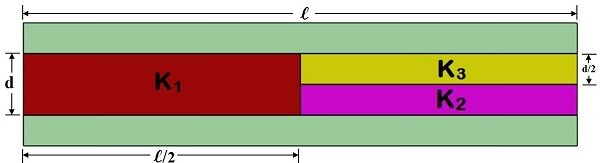Figure1 - parallel plate capacitor with three dielectrics

With the following parameters:The equivalent capacitance is:## Model

The following instructions show how to prescribe material to individual parts of your model and compute capacitance between two elements.
Model of the capacitor with multipledielectrics has been created in Solidworks. The space betweenthe parallel plates is filled by 3 different dielectric materials.Plate surface is, whilethe thickness of each plate is 1mm.Thickness of dielectric blockis the same as the distance between the plates: 2mm; thickness of blocksandis half of that:1mm (Figure 2).

The simulation is performed in the EMS Electrostatic study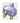. Aluminum is used as a material for the electrode plates, Teflon, polyimide and nylon are used for dielectric,and, respectively. All these materials with their electromagnetic properties can be found in the EMS material library.

### Assign material

To define material for the Dielectric 1:
1. Under Materials in the EMS manager tree, right click on the Dielectric 1.
2. Select Apply Material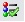3. The Material Browser folder appears
4. Under “Cables” folder, choose Teflon
5. Click Apply and Close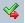This procedure is repeated to assign Polyimide and Nylon to Dielectrics 2 and 3, as well as to assign Aluminum to the plates.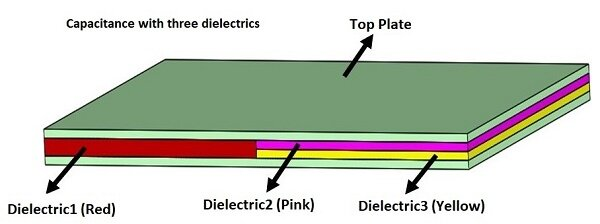Figure 2 - Solid works model of a capacitor with 3 dielectrics

### Compute capacitance

To obtain capacitance results from EMS:

1. In the EMS manger tree, Right-click on the Electrostatic studyfolder.
2. Select Properties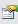3. Under General Properties, check Compute Capacitance box
4. Click OK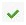.

### Boundary Conditions

To account for the capacitance, a Floating conductor boundary condition is assigned to both plates.
To do so:
1. In the EMS manger tree, Right-click on the Load/Restraint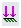folder.
2. Select Floating Conductor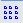.
3. Click inside the BodiesSelection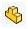box and then select the Topplate.
4. Click OK.
For the bottom plate:
1. In the EMS manger tree, Right-click on the Load/Restraintfolder.
2. Select Floating Conductor.
3. Click inside the BodiesSelectionbox and then select the Bottom plate.
4. Click OK.

## Results

In the EMS manger tree, under Results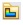, open the Results Table to find Capacitance matrix. EMS solution for total capacitance isF (Figure 3) and it matches the theoretical result very closely.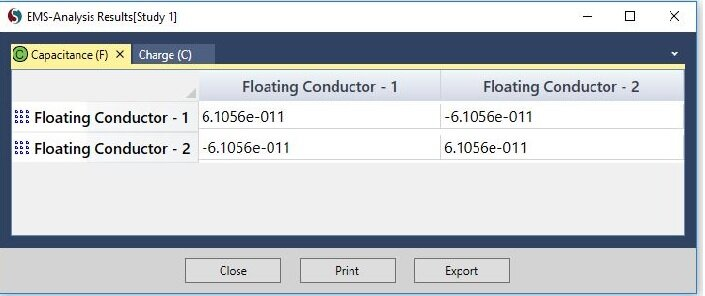Figure 3 - EMS results for Capacitance

Upcoming Webinars